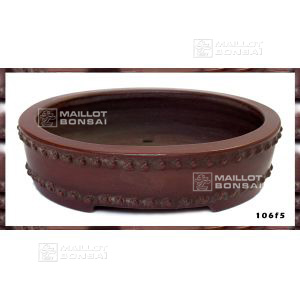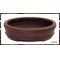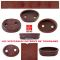##### The Japanese Bonsai specialist
Direct order Contact Help / Services Newsletter# Tokoname drum pot 106 f-1

› Bonsai pots › Tokoname bonsai potsref. : 5044

110,00

Available quantity : 3Order

###### Description

Exterior 94*70*25 mm. Interior: 80*57*16 mm. Decorated with rivets like a drum, this pot is made in the south of Japan close to Nagoya in the city of Tokoname. It's been shaped by a master potter and is stamped underneath with their seal as a mark of quality. The sandstone is very fine, making the pot pleasant to touch, and it's been fired to 1,200 degrees.

#tokoname 5.8 #drum 3.4 #bonsai 2.6 #been 2.4 #pots 2.4 #with 2.4 #underneath 2 #decorated 1.9 #sandstone 1.9 #interior 1.8

###### Technical description
 shape oval color brown origin Japan

Formule
(( ROUND((CHAR_LENGTH(b.article_nom)-CHAR_LENGTH(REPLACE(b.article_nom, 'tokoname', '')))/LENGTH('tokoname')) + ROUND((CHAR_LENGTH(b.article_description)-CHAR_LENGTH(REPLACE(b.article_description, 'tokoname', '')))/LENGTH('tokoname')) ) * 3.8) + (( ROUND((CHAR_LENGTH(b.article_nom)-CHAR_LENGTH(REPLACE(b.article_nom, 'drum', '')))/LENGTH('drum')) + ROUND((CHAR_LENGTH(b.article_description)-CHAR_LENGTH(REPLACE(b.article_description, 'drum', '')))/LENGTH('drum')) ) * 3.4) + (( ROUND((CHAR_LENGTH(b.article_nom)-CHAR_LENGTH(REPLACE(b.article_nom, 'been', '')))/LENGTH('been')) + ROUND((CHAR_LENGTH(b.article_description)-CHAR_LENGTH(REPLACE(b.article_description, 'been', '')))/LENGTH('been')) ) * 2.4) + (( ROUND((CHAR_LENGTH(b.article_nom)-CHAR_LENGTH(REPLACE(b.article_nom, 'with', '')))/LENGTH('with')) + ROUND((CHAR_LENGTH(b.article_description)-CHAR_LENGTH(REPLACE(b.article_description, 'with', '')))/LENGTH('with')) ) * 2.4) + (( ROUND((CHAR_LENGTH(b.article_nom)-CHAR_LENGTH(REPLACE(b.article_nom, 'underneath', '')))/LENGTH('underneath')) + ROUND((CHAR_LENGTH(b.article_description)-CHAR_LENGTH(REPLACE(b.article_description, 'underneath', '')))/LENGTH('underneath')) ) * 2) + (( ROUND((CHAR_LENGTH(b.article_nom)-CHAR_LENGTH(REPLACE(b.article_nom, 'sandstone', '')))/LENGTH('sandstone')) + ROUND((CHAR_LENGTH(b.article_description)-CHAR_LENGTH(REPLACE(b.article_description, 'sandstone', '')))/LENGTH('sandstone')) ) * 1.9) + (( ROUND((CHAR_LENGTH(b.article_nom)-CHAR_LENGTH(REPLACE(b.article_nom, 'decorated', '')))/LENGTH('decorated')) + ROUND((CHAR_LENGTH(b.article_description)-CHAR_LENGTH(REPLACE(b.article_description, 'decorated', '')))/LENGTH('decorated')) ) * 1.9) + (( ROUND((CHAR_LENGTH(b.article_nom)-CHAR_LENGTH(REPLACE(b.article_nom, 'pleasant', '')))/LENGTH('pleasant')) + ROUND((CHAR_LENGTH(b.article_description)-CHAR_LENGTH(REPLACE(b.article_description, 'pleasant', '')))/LENGTH('pleasant')) ) * 1.8) + (( ROUND((CHAR_LENGTH(b.article_nom)-CHAR_LENGTH(REPLACE(b.article_nom, 'exterior', '')))/LENGTH('exterior')) + ROUND((CHAR_LENGTH(b.article_description)-CHAR_LENGTH(REPLACE(b.article_description, 'exterior', '')))/LENGTH('exterior')) ) * 1.8) + (( ROUND((CHAR_LENGTH(b.article_nom)-CHAR_LENGTH(REPLACE(b.article_nom, 'interior', '')))/LENGTH('interior')) + ROUND((CHAR_LENGTH(b.article_description)-CHAR_LENGTH(REPLACE(b.article_description, 'interior', '')))/LENGTH('interior')) ) * 1.8)

## Secure payment## Delivery

Our logistic partners :04 74 55 23 48
Pépinière MAILLOT-BONSAÏ
Le Bois Frazy
01990 RELEVANT - FRANCE
on appointment